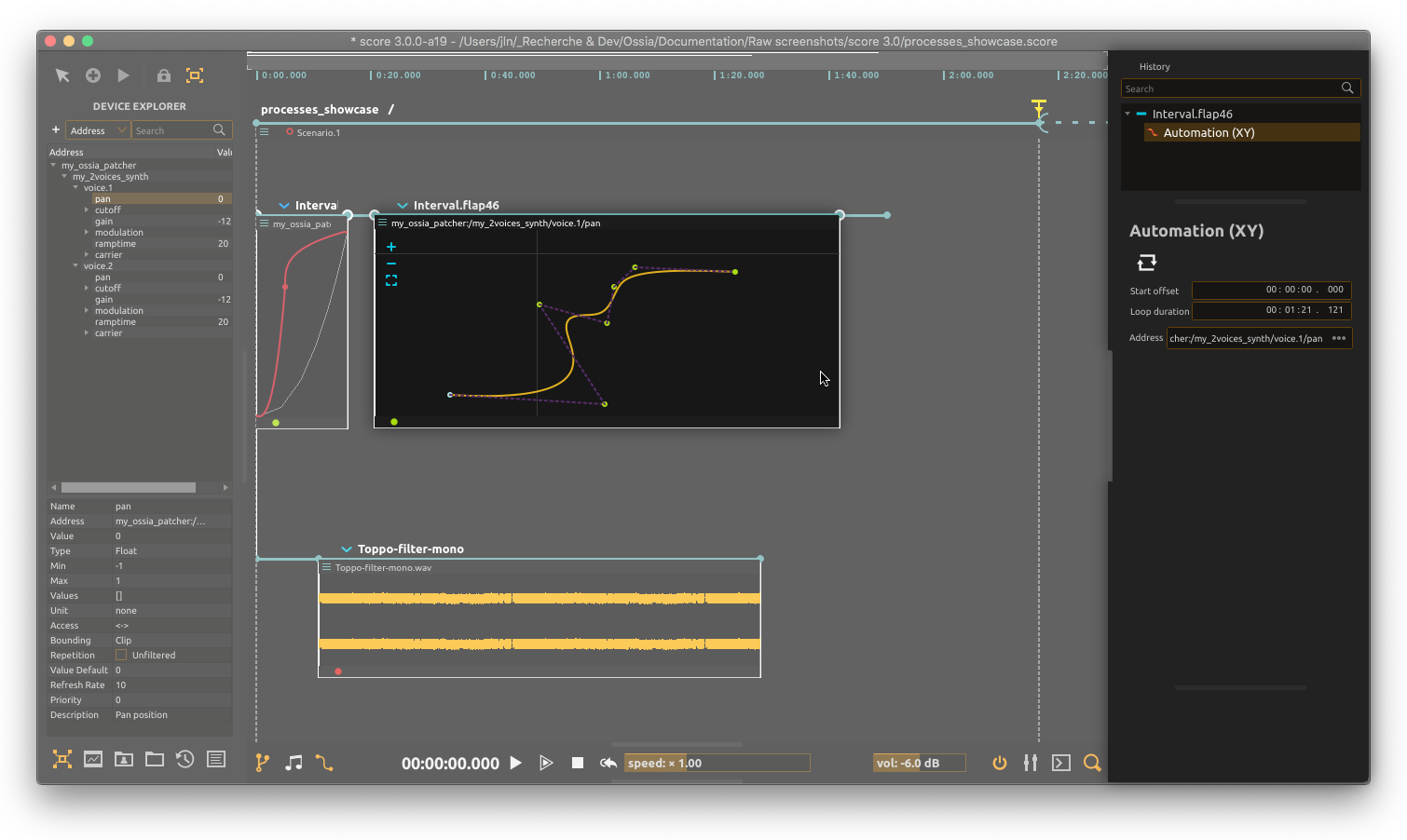# 2D Spline (X-Y automation)The 2D spline is a process which allows to control a 2D position in time, by modifying a curve in the user interface.

# Modifying the spline

Points can be dragged with the mouse.

• To create a new control point, double-click on the curve.
• To remove a point, double-click on it.
• Due to the way cubic splines work, there cannot be less than four points.

# Generating a curve

It is possible to use math formulas to generate a specific spline.

To do so, right-click on a spline, and select `Generate curve` in the menu.

The available variable is `t`. It is evaluated between 0 and 1.

The syntax uses ExprTK (see ExprTK support reference page).

Here are some useful examples:

## Circle

``````var tp := 2 * PI * t;
x := tp * cos(tp);
y := tp * sin(tp);
``````

## Spiral

``````var tp := 2 * PI * t;
x := tp * cos(tp);
y := tp * sin(tp);
``````

## Expanding spiral

``````var tp := 2 * PI * t;
x := 0.04 * exp(0.3 * tp) * cos(tp);
y := 0.04 * exp(0.3 * tp) * sin(tp);
``````

## Lissajoux

``````var tp := 2 * PI * t;
x := cos(3 * tp);
y := sin(2 * tp);
``````
``````var tp := 2 * PI * t;
x := cos(5 * tp);
y := sin(3 * tp);
``````

## Hypotrochroids

### Star 1

``````var tp := 6 * PI * t;
var a := 5;
var b := 3;
var d := 3;
x := (a - b) * cos(tp) + d * cos(tp * (a - b) / b);
y := (a - b) * sin(tp) - d * sin(tp * (a - b) / b);
``````

### Star 2

``````var tp := 6 * PI * t;
var a := 5;
var b := 3;
var d := 5;
x := (a - b) * cos(tp) + d * cos(tp * (a - b) / b);
y := (a - b) * sin(tp) - d * sin(tp * (a - b) / b);
``````

## Others

See https://en.wikipedia.org/wiki/Parametric_equation for inspiration.

``````var tp := 2 * PI * t;
var a := 4;
var b := 1;
var c := 4;
var d := 1;

var j := 3;
var k := 3;
x := cos(a * tp) - pow(cos(b * tp), j);
y := sin(c * tp) - pow(sin(d * tp), k);
``````
``````var tp := 2 * PI * t;
var a := 80;
var b := 1;
var c := 80;
var d := 1;

var j := 3;
var k := 3;
x := cos(a * tp) - pow(cos(b * tp), j);
y := sin(c * tp) - pow(sin(d * tp), k);
``````